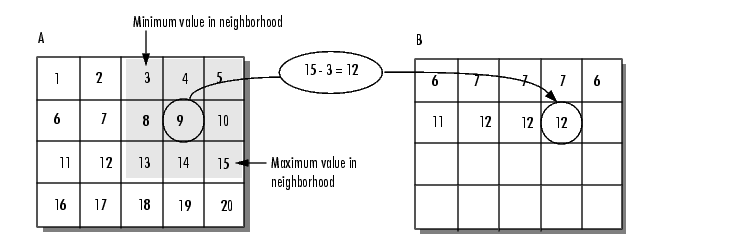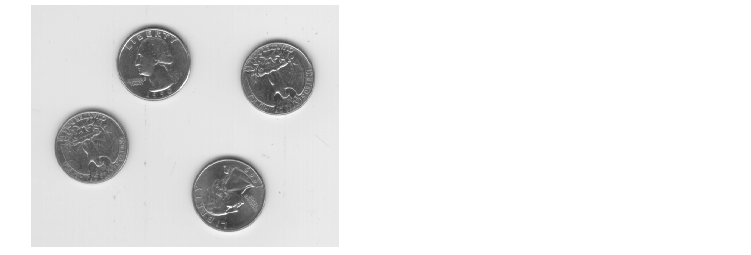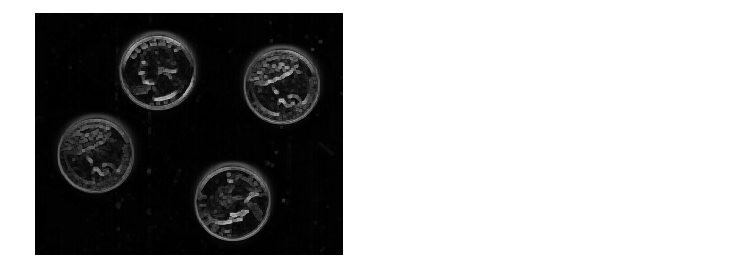Image Processing Toolbox User's GuideUsing Texture Filter Functions

The toolbox includes three texture analysis functions that filter an image using standard statistical measures, such as range, standard deviation, and entropy. Entropy is a statistical measure of randomness. These statistics can characterize the texture of an image because they provide information about the local variability of the intensity values of pixels in an image.

For example, in areas with smooth texture, the range of values in the neighborhood around a pixel will be a small value; in areas of rough texture, the range will be larger. Similarly, calculating the standard deviation of pixels in a neighborhood can indicate the degree of variability of pixel values in that region.

Understanding the Texture Filter Functions

The three statistical texture filtering functions are

`rangefilt` -- Calculates the local range of an image

`stdfilt` -- Calculates the local standard deviation of an image

`entropyfilt` -- Calculates the local entropy of a grayscale image

The functions all operate in a similar way: they define a neighborhood around the pixel of interest and calculate the statistic for that neighborhood.

This example shows how the `rangefilt` function operates on a simple array.

• ```A = [ 1 2 3 4 5; 6 7 8 9 10; 11 12 13 14 15; 16 17 18 19 20 ]

A =

1     2     3     4     5
6     7     8     9    10
11    12    13    14    15
16    17    18    19    20

B = rangefilt(A)

B =

6     7     7     7     6
11    12    12    12    11
11    12    12    12    11
6     7     7     7     6
```

The following figure shows how the value of element `B(2,4)` was calculated from `A(2,4)`. By default, the `rangefilt` function uses a 3-by-3 neighborhood but you can specify neighborhoods or different shapes and sizes.Determining Pixel Values in Range Filtered Output Image

The `stdfilt` and `entropyfilt` functions operate similarly, defining a neighborhood around the pixel of interest and calculating the statistic for the neighborhood to determine the pixel value in the output image. The `stdfilt` function calculates the standard deviation of all the values in the neighborhood.

The `entropyfilt` function calculates the entropy of the neighborhood and assigns that value to the output pixel. Note that, by default, the `entropyfilt` function defines a 9-by-9 neighborhood around the pixel of interest. To calculate the entropy of an entire image, use the `entropy` function.

Example: Using the Texture Functions

The following example illustrates how the texture filter functions can detect regions of texture in an image. In the figure, the background is smooth; there is very little variation in the gray-level values. In the foreground, the surface contours of the coins exhibit more texture. In this image, foreground pixels have more variability and thus higher range values. Range filtering makes the edges and contours of the coins more visible.

To see an example of using filtering functions, view the Texture Segmentation Using Texture Filters demo.

1. Read in the image and display it.
• ```I = imread('eight.tif');
imshow(I)```
2. Filter the image with the `rangefilt` function and display the results. Note how range filtering highlights the edges and surface contours of the coins.
• ```K = rangefilt(I);
figure, imshow(K)```Analyzing the Texture of an Image Using a Gray-Level Co-Occurrence Matrix (GLCM)